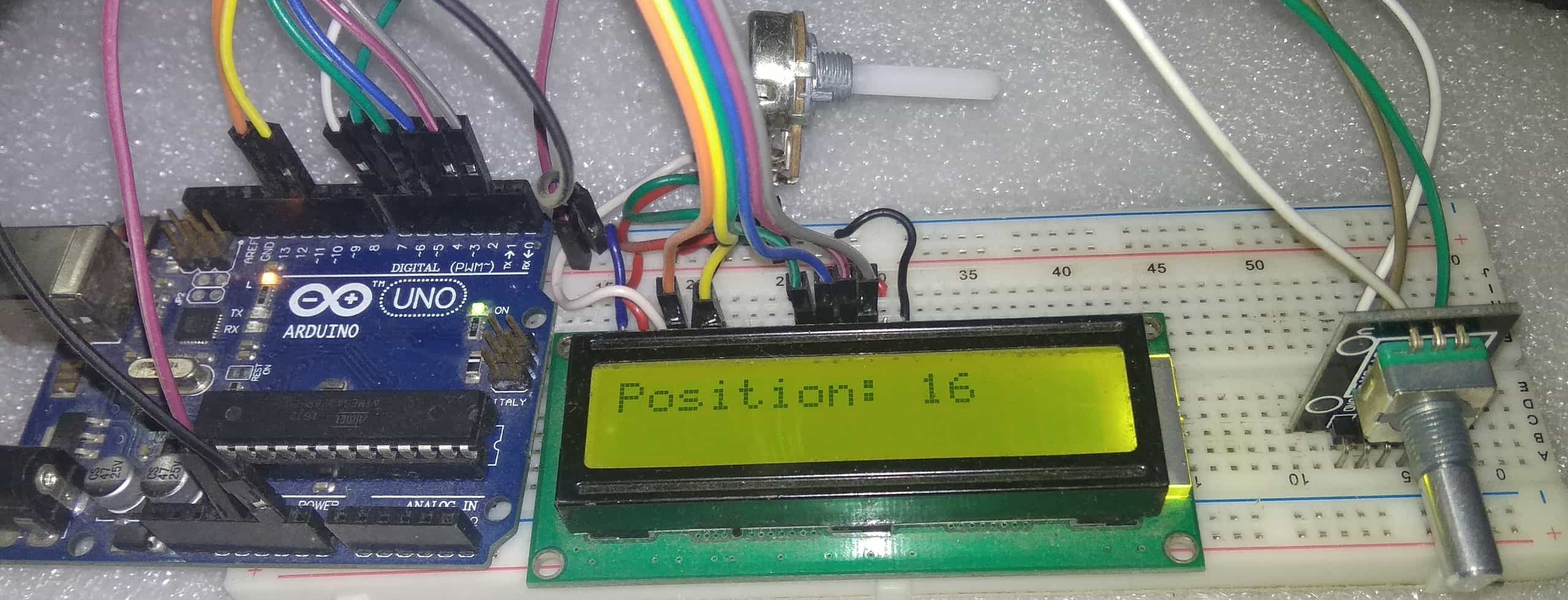How to use Rotary Encoder with Arduino(Last Updated On: March 23, 2019)

How to use Rotary Encoder with Arduino

In this post we will learn How to use Rotary Encoder with Arduino. We will be displaying the encoded value both in clockwise and anti clockwise direction in numbers with sign.A rotary encoder, also called a shaft encoder, is an electro-mechanical device that converts the angular position or motion of a shaft or axle to analog or digital output signals. There are two main types of rotary encoder: absolute and incremental. The output of an absolute encoder indicates the current shaft position, making it an angle transducer. The output of an incremental encoder provides information about the motion of the shaft, which typically is processed elsewhere into information such as position, speed and distance.

To learn about Rotary Encoder and its types with application, advantage and working visit here:

What is Rotary Encoder? Construction & Working of Rotary Encoder

Components Required:

```1. Arduino UNO Board  (Buy Online from Amazon)
2. Rotary Encoder  (Buy Online from Amazon)
3. 16x2 LCD  (Buy Online from Amazon)
4. Connecting Wires
5. 10K POT

Circuit Diagram & Connection:

The circuit diagram below is a simple demonstration on how to use Rotary Encoder with Arduino. Assemble the same circuit on breadboard or PCB.How Rotary Encoder Works?

The encoder has a disk with evenly spaced contact zones that are connected to the common pin C and two other separate contact pins A and B, as illustrated below.When the disk will start rotating step by step, the pins A and B will start making contact with the common pin and the two square wave output signals will be generated accordingly.

Any of the two outputs can be used for determining the rotated position if we just count the pulses of the signal. However, if we want to determine the rotation direction as well, we need to consider both signals at the same time.We can notice that the two output signals are displaced at 90 degrees out of phase from each other. If the encoder is rotating clockwise the output A will be ahead of output B.

So if we count the steps each time the signal changes, from High to Low or from Low to High, we can notice at that time the two output signals have opposite values. Vice versa, if the encoder is rotating counter clockwise, the output signals have equal values. So considering this, we can easily program our controller to read the encoder position and the rotation direction.

Source Code/Program:```#include <LiquidCrystal.h>
LiquidCrystal lcd(12, 11, 5, 4, 3, 2);
#define outputA 6
#define outputB 7

int counter = 0;
int aState;
int aLastState;

void setup() {
pinMode (outputA,INPUT);
pinMode (outputB,INPUT);

Serial.begin (9600);
lcd.begin(16,2);
// Reads the initial state of the outputA

}

void loop() {
// If the previous and the current state of the outputA are different, that means a Pulse has occured
if (aState != aLastState){
// If the outputB state is different to the outputA state, that means the encoder is rotating clockwise
counter ++;
lcd.clear();
} else {
counter --;
lcd.clear();
}
Serial.print("Position: ");
Serial.println(counter);
lcd.setCursor(0, 0);
lcd.print("Position: ");
lcd.setCursor(10, 0);
lcd.print(counter);

}
aLastState = aState; // Updates the previous state of the outputA with the current state

}```

Recommended For You